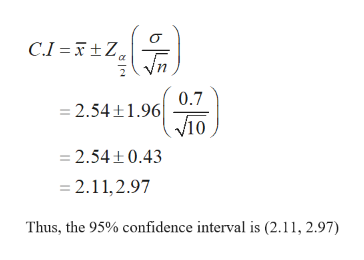Question
4 views

this is part two. I got the answer wrong and i trying to figure out the answers before this test.

Let x be a random variable that represents micrograms of lead per liter of water (µg/L). An industrial plant discharges water into a creek. The Environmental Protection Agency (EPA) has studied the discharged water and found x to have a normal distribution, with

σ = 0.7 µg/L.

Note: For degrees of freedom d.f. not in the Student's t table, use the closest d.f. that is smaller. In some situations, this choice of d.f. may increase the P-value a small amount and thereby produce a slightly more "conservative" answer.

(a) The industrial plant says that the population mean value of x is
μ = 2.0 µg/L.
However, a random sample of
n = 10
water samples showed that
x = 2.54 µg/L.
Does this indicate that the lead concentration population mean is higher than the industrial plant claims? Use
a = 1%
(i) the level of significance which is 0.01
and the value of the sample test statistic is 2.44
(iii)p-value<0.010

(iv) Based on your answers in parts (i) to (iii), will you reject or fail to reject the null hypothesis? Are the data statistically significant at level α?
At the α = 0.01 level, we fail to reject the null hypothesis and conclude the data are statistically significant.At the α = 0.01 level, we reject the null hypothesis and conclude the data are statistically significant.     At the α = 0.01 level, we fail to reject the null hypothesis and conclude the data are not statistically significant.At the α = 0.01 level, we reject the null hypothesis and conclude the data are not statistically significant.

(b) Find a 95% confidence interval for μ using the sample data and the EPA value for σ. (Round your answers to two decimal places.)
lower limit
upper limit

(c) How large a sample should be taken to be 95% confident that the sample mean
x
is within a margin of error
E = 0.4 µg/L
of the population mean? (Round your answer up to the nearest whole number.)
?  water samples

check_circle

Step 1

(a) (iv)

The null and the alternative hypotheses used for testing are given below:

Step 2

By using the results obtained in part (i) and (iii) the conclusion is made.

Decision Rule:

Reject the null hypothesis when the p-value is lesser than or equal to the level of significance. Otherwise, do not reject the null hypothesis.

Conclusion:

It is given that the p-value is lesser than 0.010 and the level of significance is 0.010.

The p-value is less than the level of significance.

Thus, the null hypothesis is rejected.

Hence, there is sufficient evidence to conclude that the lead concentration population mean is higher than the industrial plant claims at 1% level of significance.

The conclusion is that we reject the null hypothesis and conclude that the data are statistically significant

Step 3

(b)

The 95% confidence interval ...help_outlineImage TranscriptioncloseC.I =F±Z, Vn, 0.7 = 2.54+1.96 V10 = 2.54+0.43 = 2.11,2.97 Thus, the 95% confidence interval is (2.11, 2.97) fullscreen

### Want to see the full answer?

See Solution

#### Want to see this answer and more?

Solutions are written by subject experts who are available 24/7. Questions are typically answered within 1 hour.*

See Solution
*Response times may vary by subject and question.
Tagged in

### Statistics